Open in App
Not now

# How To Highlight a Time Range in Time Series Plot in Python with Matplotlib?

• Last Updated : 22 Sep, 2021

A time series plot is a plot which contains data which is being measured over a period of time, for example, a gross domestic product of a country, the population of the world and many other data.

Sometimes we want to highlight a specific period of the timeline so that it is easier for the observer to read specific data. We can highlight a time range in time series plot using the pyplot.axvspan() in matplotlib module.

Syntax:

matplotlib.pyplot.axvspan(xmin, xmax, ymin=0, ymax=1, **kwargs)

Parameters:

• xmin: Number indicating the starting position of the vertical rectangle on X-axis.
• xmin: Number indicating the ending position of the vertical rectangle on X-axis.
• ymin: Vertical rectangle starting position on y axis, it will take values between 0 and 1, 0 being bottom of the axis, 1 being top of the axis
• ymax: Vertical rectangle ending position on y axis, it will take values between 0 and 1, 0 being bottom of the axis, 1 being top of the axis.
• **kwargs: Other optional parameters to change the properties of the rectangle, like changing color etc.

Below are some examples which depict how to highlight a time range in time series plot:

Example 1:

let us assume that we have a country, and we want to draw a plot of its gross domestic product over a span of many years. Firstly we have to prepare our data so that we can plot a graph. On the Y-axis we will be taking GDP which will be random integers from 5 to 10. On the X-axis we will be taking time period from 1900 to 2020.

We will take GDP of a country and highlight it for a certain period of time.

## Python3

 `# import required modules``import` `random``import` `matplotlib.pyplot as plt` `# create dataset``year ``=` `[i ``for` `i ``in` `range``(``1900``,``2021``)]``GDP ``=` `[]``for` `i ``in` `range``(``121``):``    ``GDP.append(random.randint(``5``,``10``))``    ` `# display dataset   ``print``(``"Length of year list is: "` `+` `str``(``len``(year)))``print``(``"Length of GDP list is: "` `+` `str``(``len``(GDP)))``print``(``"First 10 elements of respective list are: "``)``print``(year[:``10``])``print``(GDP[:``10``])`

Output: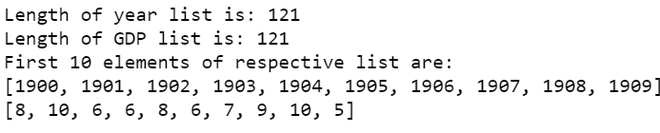Now we will plot a graph of the GDP vs year. We have scaled the Y-axis accordingly.

## Python3

 `# depict time series``fig, ax ``=` `plt.subplots(figsize``=``(``5``,``5``))``ymin, ymax ``=` `plt. ylim()``ax.plot(year,GDP)``plt.ylim(ymin ``*` `50``, ymax ``*` `50``)` `# adjust label``ax.set_ylabel(``"GDP"``)` `# assign title``ax.set_title(``"GDP of country over years"` `,size``=``15``)``plt.show()`

Output: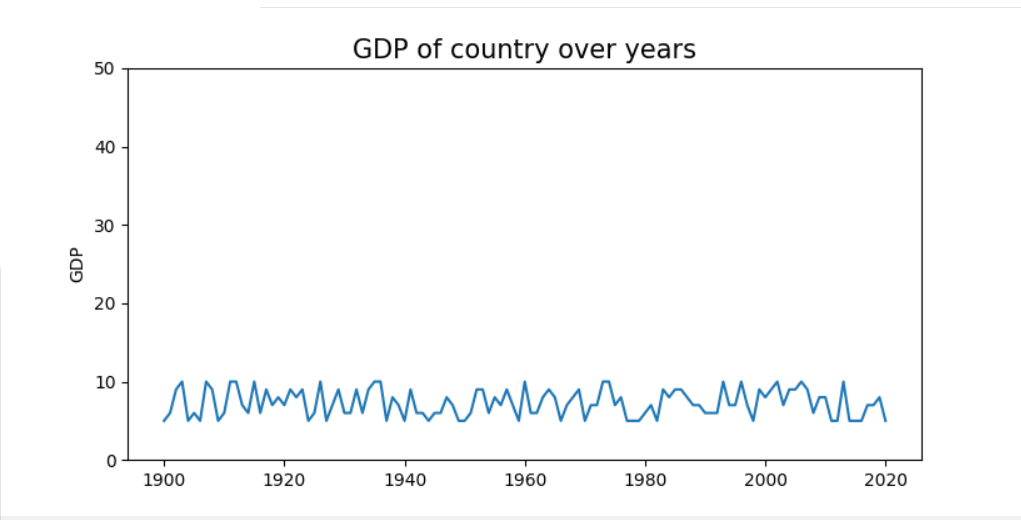graph of GDP vs year

The subplot keyword returns the figure i.e. the plot and the array of Axes (stored in ax) which we will use to highlight the graph.

We will use axvspan() method which adds a vertical span (rectangle) across the axes in a given range, and we can then change the color of the rectangle and then reduce its opacity so that it seems that we have highlighted it.

NOTE: Remember to reduce the opacity otherwise it will appear as a solid color rectangle on the graph.The  alpha argument used using **kwargs is used to reduce the opacity.

## Python3

 `# depict illustration``fig, ax ``=` `plt.subplots(figsize``=``(``5``, ``5``))``ymin, ymax ``=` `plt. ylim()``ax.plot(year, GDP)``plt.ylim(ymin ``*` `50``, ymax ``*` `50``)` `# adjust labels``ax.set_ylabel(``"GDP"``)` `# assign title``ax.set_title(``"GDP of country over years"``, size``=``15``)` `# highlight a time range``ax.axvspan(``1990``, ``2010``, color``=``"blue"``, alpha``=``0.3``)``plt.show()`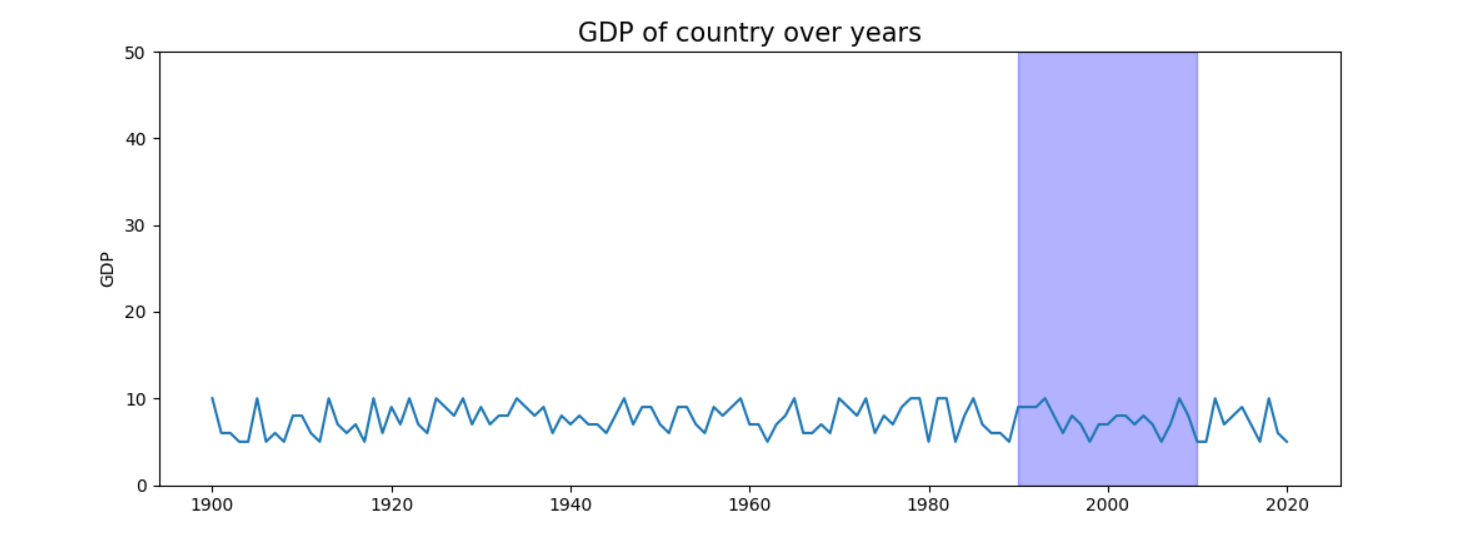highlighted graph of GDP vs year

Example 2:

Here is another program where we will highlight the x-axis for a scatter plot.

## Python3

 `# importing libraries``import` `matplotlib.pyplot as plt``import` `random` `# creating the dataset``date ``=` `[i ``for` `i ``in` `range``(``2000``, ``2021``)]``value ``=` `[]``for` `i ``in` `range``(``21``):``    ``value.append(random.randint(``5``, ``15``))` `# Create a figure and a set of subplots``fig, ax ``=` `plt.subplots(figsize``=``(``10``, ``6``))` `# Creating the scatter plot``ax.scatter(date, value)` `# Highlighting for a certain period of time``ax.axvspan(``2002``, ``2005``, alpha``=``0.3``, color``=``"green"``)``plt.show()`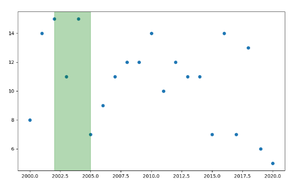highlighting over a  scatter plot

Example 3

Below is another example where we highlight a time range from 2005 to 2010

## Python3

 `# import required modules``import` `random``import` `matplotlib.pyplot as plt` `# create dataset``x ``=` `[``int``(i) ``for` `i ``in` `range``(``2000``,``2020``)]``y ``=` `[i ``for` `i ``in` `range``(``20``)]` `# depict illustration``fig, ax ``=` `plt.subplots(figsize``=``(``10``, ``10``))``ymin, ymax ``=` `plt. ylim()``ax.plot(x, y)` `# highlight a time range``ax.axvspan(``2005``, ``2010``, color``=``"green"``, alpha``=``0.6``)``plt.show()`

Output: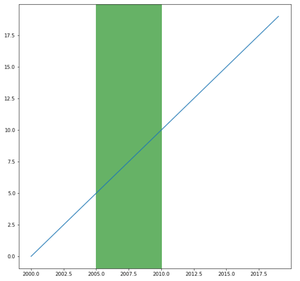My Personal Notes arrow_drop_up